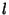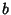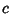## Area

The Area of a Surface is the amount of material needed to cover'' it completely. The Area of a Triangle is given by(1)

whereis the base length andis the height, or by Heron's Formula(2)

where the side lengths are,, andandthe Semiperimeter. The Area of a Rectangle is given by(3)

where the sides are lengthand. This gives the special case of(4)

for the Square. The Area of a regular Polygon withsides and side lengthis given by(5)

Calculus and, in particular, the Integral, are powerful tools for computing the Area between a curveand the x-Axis over an Interval, giving(6)

The Area of a Polar curve with equationis(7)

Written in Cartesian Coordinates, this becomes(8)(9)

For the Area of special surfaces or regions, see the entry for that region. The generalization of Area to 3-D is called Volume, and to higher Dimensions is called Content.

Gray, A. The Intuitive Idea of Area on a Surface.'' §13.2 in Modern Differential Geometry of Curves and Surfaces. Boca Raton, FL: CRC Press, pp. 259-260, 1993.